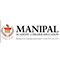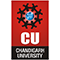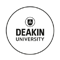Difference Between NFA and DFA

# Difference Between NFA and DFA

clickHere
Updated on Jul 31, 2023 06:46 IST

Finite Automata (FA) is the most straightforward machine to recognize patterns and can be mainly characterized into two types: NFA and DFA. You can use NFAs and DFAs in various fields related to computer science, such as formal language recognition and verification, machine learning, pattern matching, etc. But, are you aware of the difference between NFA and DFA?

The main difference between NFA and DFA is that DFA has a unique next state for each input symbol in the current state. In contrast, NFA can have multiple following states for some input symbols in the current state.

You can also explore: Curse of Dimensionality

This article will significantly explore the difference between NFA and DFA. But, before we dive deeper into the topic (Difference Between NFA and DFA), let’s go over the list of topics listed under the table of contents (TOC) that we will cover in this article.

## Difference Between NFA and DFA

For a better understanding, let’s discuss the difference between NFA and DFA in a tabular format. Here’s the table:

## What is NFA?

Definition: A Non-deterministic Finite Automata (NFA) is a type of finite automata that allows for multiple possible following states based on the current input.

In layman’s terms, an NFA is a type of finite automata that can follow multiple paths based on the input it is currently processing at any given time. The main reason it is called non-deterministic is that there is more than one possible next state for a given input.

You can also explore: KNN Algorithm in Machine Learning

Non-deterministic finite automata consist of a set of five tuples and are represented as M = (Q, Σ, δ, q0, F)

In the above representation, here’s what each tuple represents:

• Q: It represents the finite set of states
• Σ: It represents the finite set of the input symbol
• q0: It represents the initial state
• F: It represents the final state
• δ: It represents the transition function

## What is DFA?

Definition: A Deterministic Finite Automata (DFA) is a type of finite automata with a unique transition from each state to every input symbol.

In layman’s terms, a DFA is a type of finite automata that will always transition to the same next state, regardless of the current state, for any given input symbol. The main reason why it is called deterministic is that, for each input symbol, the DFA will have an actual next state.

Deterministic finite automata also consist of a set of five tuples and are represented as M = (Q, Ʃ, δ, qo, F)

In the above representation, here’s what each tuple represents:

• Q: It represents the set of final states
• Σ: It represents the finite set known as alphabets.
• q0: It represents an initial state or starts state of DFA.
• F: It represents the set of final states of DFA.
• δ: It represents a transition function defined over transitions of FA for state and input.

You can also explore: Difference Between Flowchart and Algorithm

## Key Differences Between NFA and DFA

Here are the key differences between NFA and DFA:

• NFA requires less space in comparison to DFA.
• NFA can use the Empty String transition, but DFA cannot use it.
• DFA is difficult to construct, whereas NFA is easy to construct.
• NFA stands for “Non-Deterministic Automata,” whereas DFA stands for “Deterministic Automata.”
• NFA allows for multiple possible following states based on the current input. In contrast, DFA has a unique transition from each state to every input.
• NFAs structure can be described as multiple little machines computing simultaneously. Whereas the DFAs structure can be described as a single machine.
• The number related to the next state can be one or zero in the case of NFA. Whereas the number related to the following (next) state is one in the case of DFA.

You can also explore: A Simple Explanation of the Bag of Words (BoW) Model

## Conclusion

In this article, we have discussed what NFA and DFA are. We have also discussed the difference between NFA and DFA in great detail. If you have any queries related to the topic, please feel free to send your query to us in the form of a comment. We will be happy to help.

Happy Learning!!

## FAQs

When is a string rejected by DFA and NFA?

DFA rejects a string if it terminates in a state which is different from accepting state. NFA will reject the string in the event of all branches dying or refusing the string.

Which is more powerful, DFA or NFA?

In terms of computational power, both DFA and NFA are equivalent as they both can recognize exact same set of languages which are the regular languages. However, NFA can sometimes be more expressive and simpler for certain tasks, while DFA can be more efficient for tasks that require a deterministic response.

clickHere

This is a collection of insightful articles from domain experts in the fields of Cloud Computing, DevOps, AWS, Data Science, Machine Learning, AI, and Natural Language Processing. The range of topics caters to upski... Read Full Bio

## Trending Degree Programs Courses## Top Picks & New Arrivals